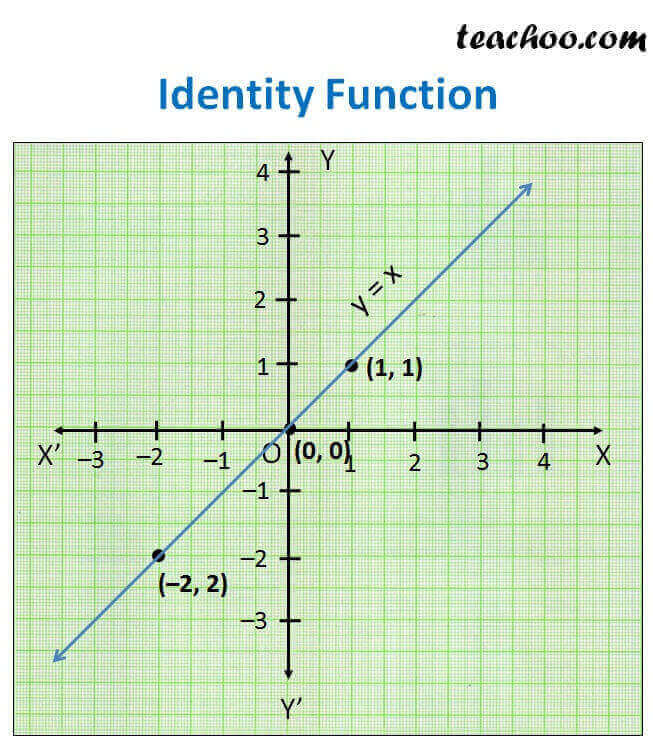Different Functions and their graphs

Chapter 2 Class 11 Relations and Functions
Concept wise

f: R -> R

f(x) = x for each x ∈ R

i.e. y = x for each x ∈ R

Plotting graph

 x 0 1 –2 y 0 1 –2Here,

Domain   = All values of x = R

Range = All values of y

= All values of x (Since x = y)

= R

Learn in your speed, with individual attention - Teachoo Maths 1-on-1 Class